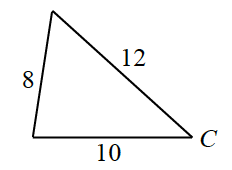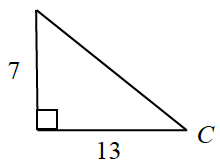### Home > INT3 > Chapter 10 > Lesson 10.1.2 > Problem10-32

10-32.

What is $m∠C$ in each triangle below?

1.Use the Law of Cosines to solve this problem.

$8^{2} = 12^{2} + 10^{2} − 2\left(12\right)\left(10\right)\text{cos}\left(C\right)$

$41.41^\circ$

1.This is a right triangle, and you know the lengths of both legs. Which trig function uses this information?